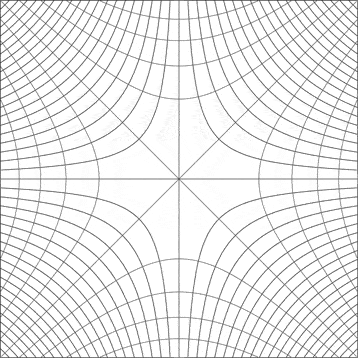# Hyperbolic coordinate system

This system of coordinates:can be "translated" in terms of x and y, so:
$$x = \sqrt{\frac{\sqrt{u^2+v^2}+u}{2}}$$
$$y = \sqrt{\frac{\sqrt{u^2+v^2}-u}{2}}$$
Exist another form more simplified of write x and y in terms of u and v? I tried rewrite these expressions using the fórmulas of half angle but didn't worked...

## Answers and Replies

Mark44
Mentor
This system of coordinates:can be "translated" in terms of x and y, so:
$$x = \sqrt{\frac{\sqrt{u^2+v^2}+u}{2}}$$
$$y = \sqrt{\frac{\sqrt{u^2+v^2}-u}{2}}$$
Exist another form more simplified of write x and y in terms of u and v? I tried rewrite these expressions using the fórmulas of half angle but didn't worked...
What about squaring each equation?
After you do that, maybe an idea will come to you.